Matplotlib Examples: Drawing Straight Lines

# Matplotlib Examples: Drawing Straight Lines

Last updated:

All code available online on this jupyter notebook

## Horizontal line

Using plt.axhline(height) is the easiest way to draw a horizontal line at a given height

import matplotlib.pyplot as plt

height = 5

plt.axhline(y=height)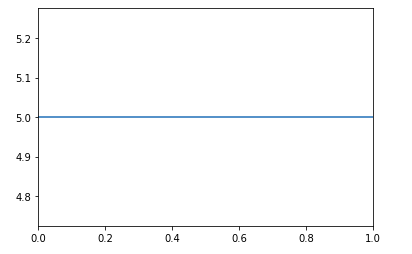Using axhline is the simplest way to draw a horizontal line in matplotlib

## Vertical line

Use plt.axvline() to draw a vertical line at a given position:

import matplotlib.pyplot as plt

x_level = 5

plt.axvline(x=x_level)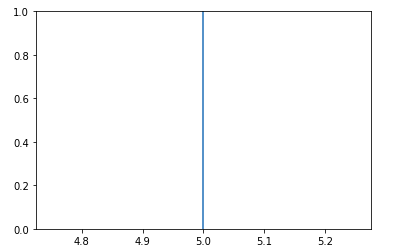Use axvline to draw a vertical line anywhere

## Vertical line, string axis

If your x-axis has strings or categories instead of numbers you can also call plt.axvline()

import matplotlib.pyplot as plt

# sample data
days   = ['sun','mon','tue','wed','thu','fri','sat']
values = [1    , 4   , 4   , 8   , 3   , 4   , 3   ]

# plotting bars for comparison
plt.bar(days, values)

plt.axvline('thu', color='red')BEFORE: a plot with string
labels in the x-axis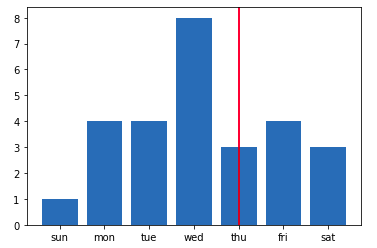AFTER: call plt.axvline('thu') to
draw a vertical line on 'Thu'

## 45 degree line passing through point

Use plt.axline(point, slope)

The slope is given in the units of the chart so you need to calcualte it based on your points:

import matplotlib.pyplot as plt
import numpy as np

# note that the x-axis is 10 times as large (in magnitude)
# as the y-axis
xs = np.random.uniform(0,100,100)
ys = np.random.uniform(0,10,100)

# just to populate the plot
plt.scatter(xs,ys,s=0.6)

# An angle of 45 degrees means a slope of 1 (relatively)
slope = (ys.max() - ys.min()) / (xs.max() - xs.min())

plt.axline((1,2),slope=slope)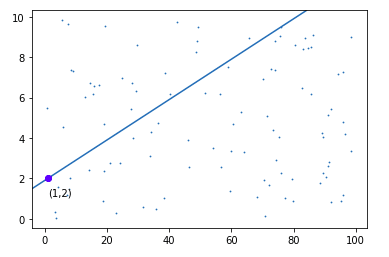We first need to calculate the slope becase it depends on
the magnitude of the axes.
Then we just call plt.axline() passing the point and the slope.

## Using Coordinates

Use plt.axline((x1,y1),(x1,y2)) to draw an infinitely long line passing through (x1,y1) and (x2,y2)

Example: Draw a line passing through (1,2) and (5,7)

import matplotlib.pyplot as plt

plt.axline((1,2),(5,7))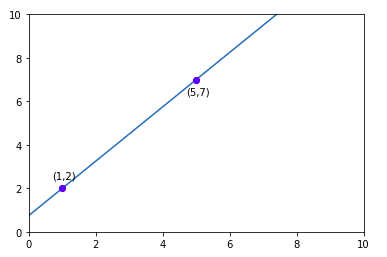Use can also use plt.axline() to draw
arbitrary lines from one point to another

## Dotted line

You can pass any of the styles here to "linestyle"

Just pass linestyle='dotted' to either plt.axvline(), plt.axhline() or plt.axline():

import matplotlib.pyplot as plt

plt.axhline(y=5,linestyle='dotted')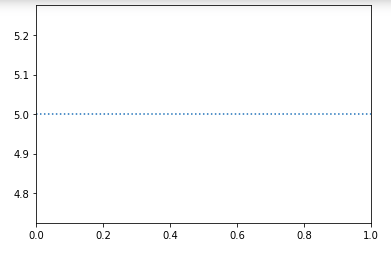Horizontal dotted line

## Dashed line

Again, everything here can be used in "linestyle"

Pass linestyle='dashed' to any of plt.axhline(), plt.axvline() or plt.axline():

import matplotlib.pyplot as plt

plt.axvline(x=5,linestyle='dashed')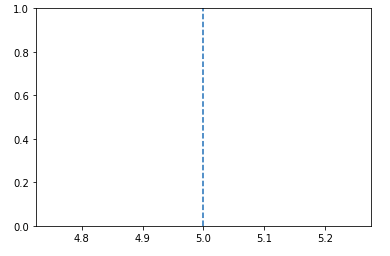Dashed line, vertical